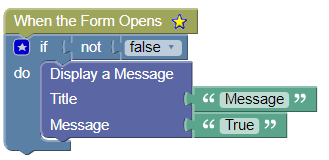# 9. Designer Logic Blocks

Home/9. Designer Logic Blocks

# 9. Designer Logic Blocks

You are here:
• 9. Designer Logic Blocks

The designer logic blocks are used to control process flow through the application. The following sections will outline each available action and provide a brief example of how the action can be used.

### IF Block

The “IF” block is used when you want to check a condition and if true then execute some additional logic.Block Configuration:

Option Description
if The condition to check
do Place any additional blocks you want executed when the “if” condition is true.

#### IF Block Example

Provided below is an example of using the “IF” block.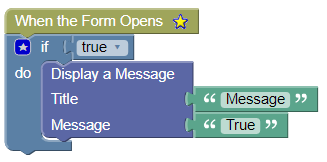### IF – ELSE Block

The “IF – ELSE” block is used when you want to check a condition and if true then execute some additional blocks and if it’s false then execute some other blocks.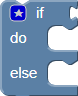Block Configuration:

Option Description
if The condition to check
do Place any additional blocks you want executed when the “if” condition is true.
else Place any additional blocks you want executed when the “if” condition is false.

#### IF-ELSE Block Example

Provided below is an example of using the “IF-ELSE” block.### IF – ELSE IF – ELSE

The “IF – ELSE – IF” block is used when you want to check a condition and if true then execute some additional blocks and if it’s false then perform another check and if that is true execute some other blocks otherwise execute some other blocks.Block Configuration:

Option Description
if The condition to check
do Place any additional blocks you want executed when the “if” condition is true.
else if The second condition to check if the first check is false.
do Place any additional blocks you want executed if the “else if” condition is true.
else Place any additional blocks you want executed when the “else if” condition is false.
Note: This is maximum condition depth that can be checked.  If you need additional checks you must structure the code in different ways.

#### IF – ELSE IF – ELSE Block Example

Provided below is an example of using the “IF-ELSE IF – ELSE” block.### Comparison Block

The “Comparison” Block is used to compare two variables and returns true or false depending on the comparison results.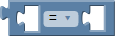Block Configuration:

Option Description
<left side> The first variable to compare
<right side> The second variable to compare
Operator “=” return true if the variables are equal, otherwise false “≠” return true if the variables are not equal, otherwise false “<” return true if the left side variable is less than the right side, otherwise false “≤” return true if the left side variable is less than or equal to the right side, otherwise false. “>” return true if the left side variable is greater than the right side, otherwise false “≥” return true if the left side variable is greater than or equal to the right side, otherwise false

#### Comparison Block Example

Provided below is an example of using the “Comparison” block.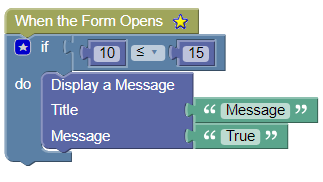### Logical Comparison Block

The “Logical Comparison” Block is used to compare two variables and returns true or false depending on the logical comparison results.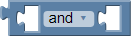Block Configuration:

Option Description
<left side> The first variable to compare
<right side> The second variable to compare
Operator “and” return true if both variables are true, otherwise false “or” return true if either variable is true, otherwise false

#### Logical Comparison Block Example

Provided below is an example of using the “Logical Comparison” block.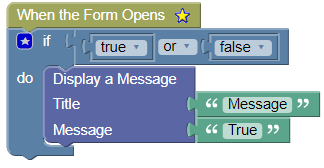### Not Block

Use the “Not” block to reverse/negate a Boolean variable.  This block must be used in conjunction with another variable.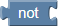Block Configuration:

Option Description
<input> The variable to negate

#### Not Block Example

Provided below is an example of using the “Not” block.